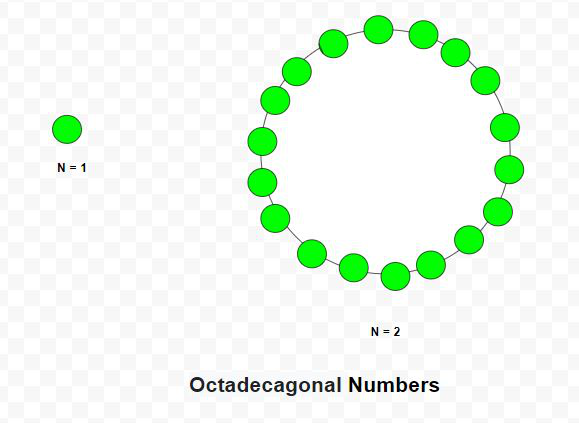Related Articles

• Last Updated : 18 Mar, 2021

Given a number N, the task is to find the Nth Octadecagonal number.

An Octadecagonal number is a class of figurate numbers. It has 18 – sided polygon called Octadecagon. The Nth Octadecagonal number count’s the eighteen number of dots and all other dots are surrounding with a common sharing corner and make a pattern. The first few Octadecagonal numbers are 1, 18, 51, 100, 165, 246, 343 …

Examples:

Input: N = 2
Output: 18
Explanation:
The second octadecagonal number is 18.
Input: N = 6
Output: 246Approach: The Nth Octadecagonal number is given by the formula:

``

Below is the implementation of the above approach:

## C++

 `// C++ program to find Nth``// Octadecagonal number` `#include ``using` `namespace` `std;` `// Function to find N-th``// Octadecagonal number``int` `Octadecagonal_num(``int` `n)``{``    ``// Formula to calculate nth``    ``// Octadecagonal number``    ``return` `(16 * n * n - 14 * n) / 2;``}` `// Driver code``int` `main()``{``    ``int` `n = 3;``    ``cout << Octadecagonal_num(n) << endl;` `    ``n = 10;``    ``cout << Octadecagonal_num(n) << endl;` `    ``return` `0;``}`

## Java

 `// Java program to find Nth``// octadecagonal number``import` `java.io.*;``import` `java.util.*;` `class` `GFG{``    ` `// Function to find N-th``// octadecagonal number``static` `int` `Octadecagonal_num(``int` `n)``{``        ` `    ``// Formula to calculate nth``    ``// octadecagonal number``    ``return` `(``16` `* n * n - ``14` `* n) / ``2``;``}``    ` `// Driver code``public` `static` `void` `main(String[] args)``{``    ``int` `n = ``3``;``    ``System.out.println(Octadecagonal_num(n));``    ` `    ``n = ``10``;``    ``System.out.println(Octadecagonal_num(n));``}``}` `// This code is contributed by coder001`

## Python3

 `# Python3 program to find Nth``# octadecagonal number` `# Function to find N-th``# octadecagonal number``def` `Octadecagonal_num(n):``    ` `    ``# Formula to calculate nth``    ``# octadecagonal number``    ``return` `(``16` `*` `n ``*` `n ``-` `14` `*` `n) ``/` `2` `# Driver code   ``n ``=` `3``print``(``int``(Octadecagonal_num(n)))` `n ``=` `10``print``(``int``(Octadecagonal_num(n)))` `# This code is contributed by divyeshrabadiya07    `

## C#

 `// C# program to find Nth``// octadecagonal number``using` `System;` `class` `GFG{``    ` `// Function to find N-th``// octadecagonal number``static` `int` `Octadecagonal_num(``int` `n)``{``        ` `    ``// Formula to calculate nth``    ``// octadecagonal number``    ``return` `(16 * n * n - 14 * n) / 2;``}``    ` `// Driver code``public` `static` `void` `Main(``string``[] args)``{``    ``int` `n = 3;``    ``Console.Write(Octadecagonal_num(n) + ``"\n"``);``    ` `    ``n = 10;``    ``Console.Write(Octadecagonal_num(n) + ``"\n"``);``}``}` `// This code is contributed by rutvik_56`

## Javascript

 ``
Output:
```51
730```

Attention reader! Don’t stop learning now. Get hold of all the important DSA concepts with the DSA Self Paced Course at a student-friendly price and become industry ready.  To complete your preparation from learning a language to DS Algo and many more,  please refer Complete Interview Preparation Course.

In case you wish to attend live classes with experts, please refer DSA Live Classes for Working Professionals and Competitive Programming Live for Students.

My Personal Notes arrow_drop_up